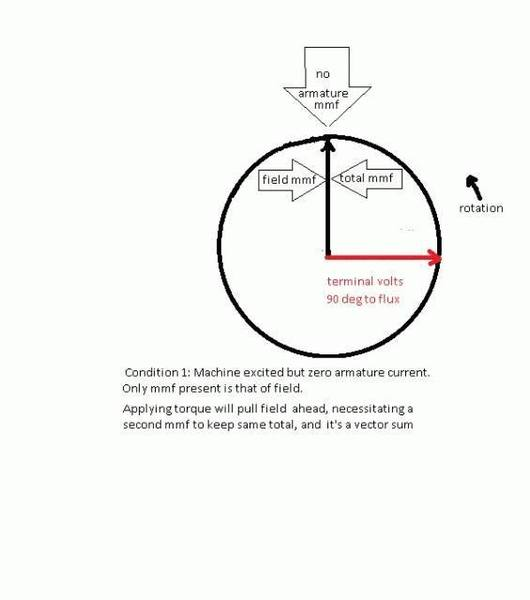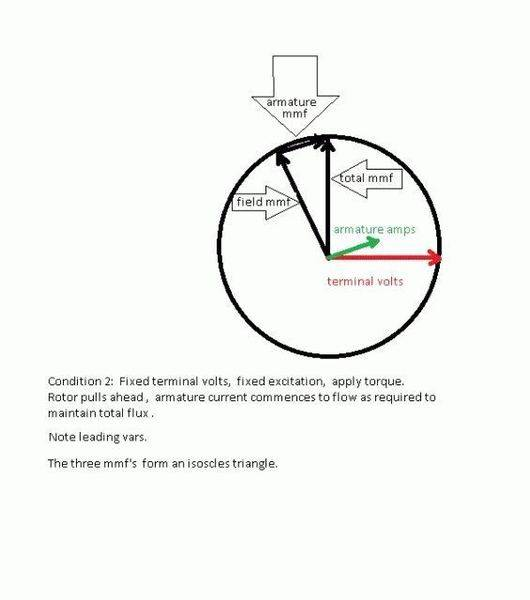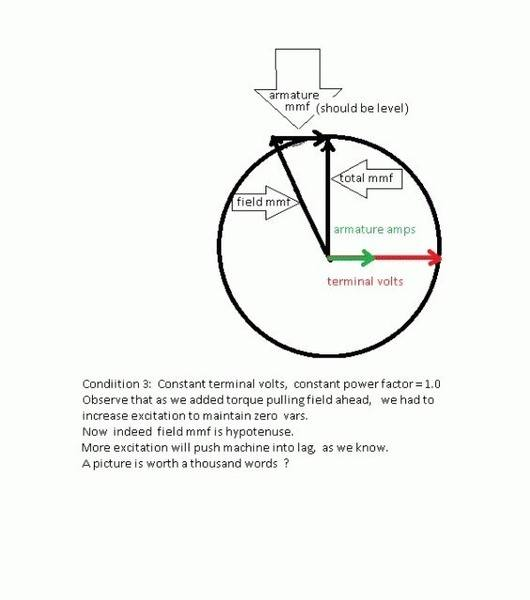# Voltage and Reactive Power Relationship

Hi,

I have some trouble regarding the relationship between voltage and reactive power. From what I've read you control the amount of reactive power the generator is producing or absorbing by increasing or lowering the excitation of the generator.

I have some trouble getting my head around this, so I was wondering if someone could show me the equation that shows me when I increase the excitation I increase the amount of reactive power into the grid and vice versa.

I need to see the equation to see how this relates, thats how my brain is put togheter, or if someone could attempt to explain this to me I would be glad.

Thank you for any help, best regards.

anorlunda
Staff Emeritus
There are two approaches to answering your question. Grid centric or generator centric.

The grid centric approach is explained in the PF Insights article https://www.physicsforums.com/insights/ac-power-analysis-part-2-network-analysis/. VAR flow is proportional to voltage differences. Raising field voltage raises terminal voltage, thus sending more positive VARS to the grid.

In the generator centric view, how does field voltage relate to terminal voltage? Those are called Park's Equations. See http://electrical-riddles.com/topic.php?lang=en&cat=5&topic=390

•cnh1995 and OliskaP
jim hardy
Gold Member
Dearly Missed
the key is to understand the machine is mostly inductive
and it sums mmf's

drawing phasor diagrams will help.....
assume machine is tied directly to infinite bus so terminal voltage is fixedwe have control of the torque applied to the shaft
and control of the field current
let's vary them one at a timenext i'll raise excitation just enough to bring power factor back to 1.0I am equation challenged so think in pictures,
sorry if above seems over-simplistic but it's how i figure out the formulas.

Think of the generator as an ideal voltage source with voltage in proportion to field current,
and an inductance in series equal to Zsynchronous which is a physical characteristic of the machine..

i hope above helps you accept the math.

•OliskaP
Both of you, thanks, and jim for taking the time creating the drawings.

...

By the way jim hardy, will the total MMF be constant? From what I see from the pictures, you adjust the field MMF by adjusting the field current, so will the armature MMF "adjust automatically" to maintain constant total MMF?

jim hardy
Gold Member
Dearly Missed
so will the armature MMF "adjust automatically" to maintain constant total MMF?

Remember i postulated connection to infinite bus.
Yes is the answer, with that qualification. Ohm's law dictates it.
Armature current will flow and remember that mmf is current X number of turns.

I further simplified by omitting non-liearity of iron, i used mmf and flux interchangeably.
Voltage is proportional to flux
Omitting the nonlinearity of BH curve simplifies the explanation. I think that's necessary to get that "intuitive feel" for the synchronous machine.
Sans that nonlinearity voltage would be linear with total MMF . You'll see something called "Potier Triangles" that helps adjust calculations for nonlinearity.

The effect is called "Armature Reaction" and "Synchronous Impedance" ..
You'll study both soon in your coursework i'm sure.

If you add impedance between machine terminals and infinite bus , then of course terminal volts will change.

•OliskaP
In that phasor diagram observe that total mmf must be perpendicular to terminal volts, and must have same radius..

Why must the terminal volts and total MMF have the same radius? I understand that terminal volt should be of a constant value if connected directly to infinite bus, but I do not fully understand why the total MMF should be of the same magnitude/radius as terminal voltage?

jim hardy
Gold Member
Dearly Missed
Why must the terminal volts and total MMF have the same radius? I understand that terminal volt should be of a constant value if connected directly to infinite bus, but I do not fully understand why the total MMF should be of the same magnitude/radius as terminal voltage?

two ways to explain what i did
1.
I used MMF and Fux interchangeably , ignoring nonlinearity of iron. That's a simplification.
If you allow that departure from rigorous math
then mmf NI and flux Φ are linearly proportional
because Φ = μμ0NIArea/Length
only non-constant in that formula is current I

Now since voltage is proportional to flux* , it is also proportional to MMF
(* Faraday's law, e = ndΦ/dt and since d(sinωt )= ωcosωt they're proportional by ratio ω)
choice of radii is up to the presentor and i chose to keep the presentation as simple as i could.

2.
Power engineering uses "Per Unit" notation where everything is calculated as a ratio to some base,
usually machine ratings for an individual machine or 100mva for power system problems.

So i , without explaining it first,
I started with terminal voltage at 1.0 of nominal rating, so set radius = 1.0 , 1 per unit volts
field amps at 1.0 of whatever is required to make 1.0 nominal rated volts at rated rpm and unloaded , 1 per unit field amps
field mmf then is 1.0 of whatever are field amps X number of turns on field, for simplicity i set turns = 1 and used same radius = 1.0 per unit field mmf
(though you'll never find field mmf on a machine's nameplate)

then i rotated field mmf some arbitrary amount ccw to represent power angle at some applied torque
drew the phasor that completed the isoscles triangle

for armature amps i just lifted that phasor, changed its color and pasted ot at the origin because that's what armature amps do, flow as necessary to make total flux match terminal volts..

What's set by physics is that terminal volts and total flux must be perpendicular late edit- and in proportion
if you want to redraw the pictures with different radii for mmf and terminal volts go ahead , I drew mine using plain old windows "Paint"
but i don't see a need for that when you're just trying to get the mental models working in your head.

.................................................................................

all that said,
••